# Perimeter Magic Polygons

Last time we looked at the classic puzzle of magic squares. Many questions we get are about similar kinds of puzzles, and here I want to look at “magic polygons” (triangles, squares, pentagons) in which, unlike the traditional magic squares, only the edges count. These are a common subject of elementary-level questions.

## Four sides: corners and parity

First is a question from 2002 about squares:

Eight Circles, Sum is 13

Place the numbers 1,2,3,4,5,6,7,8 in the eight circles so that the sum of the numbers in any line equals 13.

O     O     O

O           O

O     O     O

There are two different solutions.

O     O     O

O           O

O     O     O

I am getting nowhere. Please help soon.

So we want something like this, which is less than a full magic square, and therefore more flexible: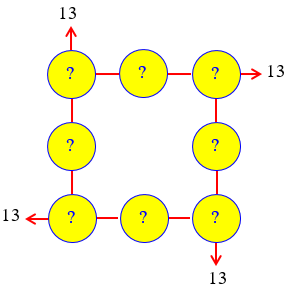Doctor Greenie took this:

Hi, Trevor -

Thanks for sending this nice little puzzle to us here at Dr. Math.  I had some fun and got some good mental exercise in reasoning this one out.

The given information says the sum of the numbers in each row of three must be 13. The total of all four of these rows is then 13*4=52.

The sum of the numbers we are to use: 1, 2, ..., 7, and 8, is 36.

In finding the sum of the four rows of three, the numbers in the corners of the array of circles get counted twice. This means that the difference between the sum of the numbers in all four rows of three and the sum of the eight numbers we are using is the sum of the four numbers in the corners of the array.

So we know the following:

the sum of the four corner numbers is 52 - 36 = 16

The four corners together account for the difference between the sum of the 8 numbers, and the sum of the four sums, so they must add up to 16: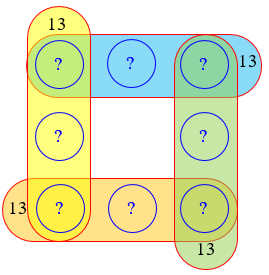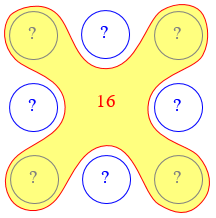Now, with the sum of the three numbers in each row being the odd number 13, each of these four rows must contain either (a) three odd numbers or (b) two even numbers and one odd number.  Since the whole array must contain four even and four odd numbers, the only possible arrangements of evens and odds in the array are the following two (where "E" and "O" denote even or odd numbers, respectively):

E   O   E        O   O   O

O       O   or   E       E

E   O   E        E   O   E

One way to see this is to start with the lower left number. If it is even, then exactly one other number in its row can be even, and likewise in its column. If the lower right is even, then depending on whether the upper left is even we are forced into the first arrangement or the second by the need for four of each. If the lower left were odd, either its row is all odd or both others are even, and we are forced into one of these, both of which are equivalent to the second pattern:

    E   O   E        O   E   E

E       E   or   O       O

O   O   O        O   E   E

This sort of thinking about “parity” (whether a number is even or odd) is often useful to restrict options.

However, we can rule out the first possibility [even corners], because in that configuration, the sum of the four corner numbers is 2+4+6+8=20; and we have determined that the sum of the four corner numbers must be 16.

So the configuration of even and odd numbers in the array is the second pattern shown above.

So now we know that one row is all odd. And we can be sure which numbers those are, since 1+3+5+7 = 16, so it must be the 3 that is omitted there. Doctor Greenie went a different direction:

Now, the sum of the numbers in the two vertical rows must be 13*2=26; and the sum of the four even numbers is 20; therefore, the sum of the two odd numbers in the two vertical rows must be 6, so those odd numbers are 1 and 5.  And then the other odd number in the top row must be 7 to make the sum in that row 13; so the odd number in the bottom row is 3.

So now we have determined that the configuration is

1   7   5

E       E

E   3   E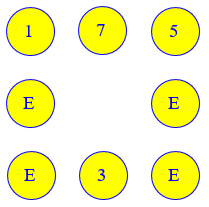From here, you can determine the possible placements of the even numbers in their four slots by working with the required sum of 13 in either vertical row or in the bottom row; you will see that there are two solutions in the pattern we have established:

1   7   5          1   7   5

4       6    or    8       2

8   3   2          4   3   6

These are the two solutions to your problem.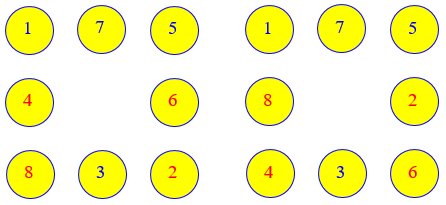All solutions can be obtained from these by rotation or reflection, for a total of 16.

## Transforming a problem

A 2004 question took a challenging problem and made it harder:

Making a Hard Square Sum Problem Easier

My name is Danny and I am in 6th Grade.  We are working on fractions and I have been given the following problem to solve.

Given these 8 numbers:  1/4, 1/2, 3/4, 1, 1 1/4, 1 1/2, 1 3/4, and 2, I am to place 3 numbers along each side of a square so that the sum of the 3 numbers on each side of the square is equal to 3.

It seems like I have tried every combination but when I get to the fourth side the 3 remaining numbers don't add up to 3.  Is there a trick to doing this kind of problem?  I really would appreciate some help.  Thanks!

I answered this question, not by working directly with the ugly numbers given, but by transforming the problem to a nicer one (which may remind you of a trick we used last week):

I suspect you are supposed to just do a lot of trial and error, giving you lots of practice adding fractions.  But there are some tricks that I used to solve it--mathematicians are always looking for easier ways to solve problems!

One trick is to avoid fractions entirely.  Notice that all your fractions are multiples of 1/4.  What would happen if we had a solution to your problem, and then multiplied every number by 4?  It would still have the sum of each side the same, but those sums would be 4 times as much.  So we can solve your problem by first solving an easier problem:

Arrange the numbers 1,2,3,4,5,6,7,8 around a square so that the sum of the three numbers on any side is 12.

O + O + O = 12
+       +
O       O
+       +
O + O + O = 12
=       =
12     12

The numbers in the solution to this problem will all be 4 times those in the other, so dividing them by 4 will give the solution. The whole thing could have been done using the fractions (which I would write using their common denominator, to make adding them easier, at least); but anything that will reduce the chances of error, and make the problem feel more tractable, is good.

From there, I moved on to the trick used above, finding the sum of the corner numbers:

That will take a lot less arithmetic to figure out; but there's still another trick to use.  Think about what happens when you add all four sides together: the sum will be 4 times 12, or 48; but you will have added the corners in twice and the side numbers only once.  That is,

sum of all eight numbers + sum of corners = 48

Do you see that?  From this you can figure out what the sum of the corner numbers has to be, and there are only a few ways that can happen.  So you have only a few possibilities to try.

Since the sum of the numbers 1 through 8 is 36, the sum of the corners must be 12. They can only be 1+2+3+6, or 1+2+4+5.

Once you solve that, you can change your solution into a solution of the original problem.  Think about what we did to change it from the fractions to the whole numbers, and reverse that process to go from the whole numbers back to the fractions.

This is an example of how mathematical thinking can change a hard problem into an easier one.  And that's what makes math a fun challenge--it's no fun to do a lot of arithmetic, but it's fun to find sneaky ways to avoid it!

We’ll finish the problem as part of the next answer.

## Sums of 12

One Box of Eight Digits, Four Sums of Twelve

Place the numbers 1, 2, 3, 4, 5, 6, 7, 8 along the outside of a 3x3 grid so that the sum of the numbers in each of the two rows or columns equals 12. Each of the numbers 1-8 can only be placed once.

So far, I've figured out that the 8 must appear with the 1 and the 3. I've tried to use that discovery to arrange the other five digits -- and nearly get all four edges to total 12 -- but keep running into a wrong sum along the way, as in the left edge of this array:

1   8   3
5       7
4   6   2

Please help. I'm 42, studying to become a educational assistant. This was an example we were given in one of my courses for fun. I'm so lost and am getting frustrated as I haven't been in school forever.

Shannon has clearly done some good initial work. I answered:

I'm glad this was given to you "for fun"; these things can be frustrating if you feel you have to do them. But learning to meet the challenge can also be exhilarating. (Well, maybe that's too strong a word for most people. But at least, learning that you can control the frustration by looking for ways to reduce the complexity of the problem is a valuable outcome of doing these -- and one that you can then pass on to kids.)

I’m always encouraged when a puzzle is presented in the right way. And I love helping future (or current) teachers.

I chose to do what Shannon must have done (thinking about what sums can make 12), and see what more can be done with that:

You've made a good start by noticing that 8 can only occur with 1 and 3; that means 8 has to be in the middle of a side, since it can't be shared by two sums.

I haven't worked out an answer yet, but am just thinking out loud here: why not continue your same line of reasoning, and see if any other numbers can fit only one way? That may lead to wondering if it would help to make a list of ALL ways to add three different numbers to get 12.

Let's start listing:

1 3 8
1 4 7
1 5 6
2 3 7

I think we're in luck; this won't be a long list. (Do you see the method I'm using to make an orderly list?)

I wanted to leave as much as possible for Shannon to do; but here is the full list, as I showed it above:

1     + 3                 + 8 = 12
1         + 4         + 7     = 12
1             + 5 + 6         = 12
2 + 3             + 7     = 12
2     + 4     + 6         = 12
3 + 4 + 5             = 12

Continuing to Shannon,

Finish this, and then maybe look for a group that uses the 1 (for the left side), a group that uses the 3 (for the right side), and one more triple that can go on the bottom. This will be more like assembling a small jigsaw puzzle than tackling a huge task by trial and error.

Now, I'll step aside and try actually solving it with this insight, to make sure I'm not leading you in a wrong direction or missing a really nice trick.

... and it worked just fine!

I often make suggestions without actually solving a problem first, in order to be working with them rather than for them; but when I do that, sometimes I miss an issue that will stump them. This time, there was no problem with my plan.

Here’s how that method worked: We have the top,

3   8   1

There are two possibilities for the column under 3 (2 and 7, or 4 and 5), and two for the column under 1 (4 and 7, or 5 and 6). Since the two columns can’t share any numbers, they must be {2, 7}{5, 6}. Now, the number in the middle of the bottom must be the one unused number, 4; and to make the bottom row sum to 12, the other numbers must be 2 and 6. Our answer is:

3   8   1

7       5

2   4   6

### Corners and parity: reprise

This was a strongly trial-and-error method. What if we had used Doctor Greenie’s method on this one?

First, what is the sum of the corner numbers? The four sides add up to 48, which is 12 more than the sum of the numbers, so the corner numbers must add up to 12. They must be either 1 + 2 + 3 + 6 or 1 + 2 + 4 + 5.

How about parity? Each side, having an even sum, must be either all even, or one even and two odds. The only possible patterns are

O   E   O        O   E   O

O       O   or   E       E

E   E   E        O   E   O

Now we can list the possible sums to 12, as we did with full magic squares:

With three evens in a row, we must have (in some orientation)

3   8   1

7       5

2   4   6

With odds in the corners, the sum of the corners would be 16, not 12. So there is only one solution (ignoring rotations and reflections), which is the same one we found by trial and error.

### Back to fractions

Finishing up Danny’s problem, we have to divide everything by 4:

3/4 8/4 1/4     3/4  2  1/4

7/4     5/4  =  7/4     5/4

2/4 4/4 6/4     1/2  1  3/2

We never heard back from Danny to be sure how he did.

## Magic Pentagon

Here is a question from 2001:

Magic Pentagon

There is a pentagon, and on each side of the pentagon, there are three circles. How can you make the sum of the three circles all the same as the others? You can only have 4 answers: 14, 16, 17, 19. You can only use the numbers from 1-10, and you can only use each of them once because there are only 10 circles.

I figured out all the possible three numbers that could add up to 14.  None of them are the same, so it looks as if there are only a few.

1 + 3 + 10 = 14
1 + 4 +  9 = 14
1 + 5 +  8 = 14
1 + 6 +  7 = 14

2 + 3 +  9 = 14
2 + 4 +  8 = 14
2 + 5 +  7 = 14

4 + 3 +  7 = 14

5 + 3 +  6 = 14

I looked in the archive and found a similar question but I still can't figure this one out.

Note that here we are given a list of magic sums; we could have worked out what the possibilities are, but they’ve saved us that work.

Ramona has already done some of the work I’d have suggested!

I had a few more ideas, starting with the old corner trick:

I can suggest a few tricks that help a lot in problems like this. I easily found the solution where the sums are 14 using these methods, and you can probably do the rest almost as easily.

First, what happens if you add all five sides together? The sum will be 5*14, or 70; but that will be the same as adding all ten numbers, and then adding the five corner numbers again (since each of them counts on two sides). This says that 55 (the sum of 1 through 10) plus the sum of the corner numbers is 70; so the corner numbers add up to 70-55, which is 15. A little thought will show you exactly which numbers you have to use at the corners!

Now, place the first of those numbers (1) at one corner, say the top. You will find that the second number (2) can't go next to the 1, because then the number between them would have to be 11 in order to get a sum of 14. So you can place the 2 at one of the bottom corners. Now where can the third number go? If it is next to the 2, you will find a problem, so that leaves only one place for it. Keep on like this to fill in the corners.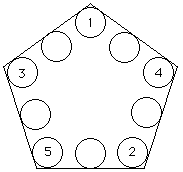Now just find what has to go in the middle of each side in order to add up to 14, and see if it works out.

I recommend trying the sum of 19 next, before tackling what are probably the hardest cases, 16 and 17. But I haven't tried them myself yet, so maybe you'll find they're easy!

This puzzle is probably meant mostly to give you lots of practice adding, but also lets you develop your own ways to solve logical problems. It won't all be easy, but at the least you will learn how to go through all the possible arrangements in an orderly way. At best, you may find an even better way to solve these than I came up with!

I’ll leave it to you to solve the other cases.

We had a similar question the next year:

Pentagon Puzzle

We have also been asked about magic triangles of the same sort, typically asking a different kind of question:

Magic Triangle Sums

Stay tuned for more puzzles, from time to time.

This site uses Akismet to reduce spam. Learn how your comment data is processed.